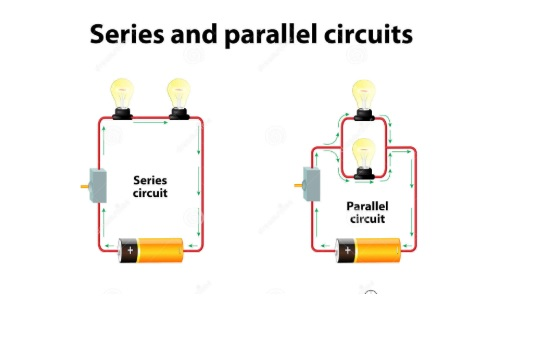# Combination of Resistors – Series and Parallel### Introduction

What do you do when you have a very large water source, say like a river or canal and you want to give water to a small field? Well, if you simply leave the field open to the river or the canal, you would simply submerge and be rendered unusable for any purpose, such as irrigation. Therefore, the most viable and successful option is to connect the field through a restricted channel that offers resistance to water flow, so that only a lot of water enters the field, since it can be handled safely without suffering damage.You may wonder what this has to do with electrical or electronic technology, but here is the trap. If you have a large power source and want to feed a smaller component, you need to divide the current or voltage as needed. This is done through the use of appropriate electronic circuits of which the resistors are an integral part. So let’s see in the next section how the resistors and their various combinations are used to alter the voltage and current in the circuits

### The Series Arrangement

It is said that the resistors are connected in series arrangement when they are joined with one another so that the current flows from the first resistance and between the second, and so on. Suppose there are 3 resistors (this is just a random number, the rules described here also apply to more or fewer numbers) that have values R1, R2 and R3 ohms respectively.

Now, if these resistors are connected in series, then the total resistance of their combination is the sum total of their individual resistances that can be written mathematically as follows

R = R1 +R2 + R3

Where R is the total resistance

Other characteristics of this arrangement are that the current remains the same in all resistors obviously because it has only got a single path to flow but the potential difference or the voltage across each resistor varies in proportion to the size of the resistor

If you remember the equation V=I*R

Then if the current remains the same (as in case of series arrangement), the voltage varies proportionately to resistance. This means that the bigger resistor would have a greater drop across it and vice versa.

In series connection current remains the sameA common application of series circuit in consumer electronics is in batteries, where several cells connected in series are used to obtain a convenient operating voltage.

### The Parallel Arrangement

Sometimes it must be so required that the voltage required might be the same across different sections but current requirements are different. Hence in such a case the resistors are not joined end to end but in parallel so that one end of all resistors is joined together at one side, while the other ends join together on the other side and this obviously is known as the parallel arrangement.

In this case the current has now three different paths to flow so it gets divided in proportion to the ease with which it can flow into each resistor. This means that the greater resistance would have smaller current flowing across it and vice versa. There is another difference here that the voltage across all resistors is the same unlike the parallel arrangement described in the previous section.

The total resistance R in such a case is given by

1/R = 1/R1 + 1/R2 + 1/R3

In parallel connection voltage remains same ie why we use parallel connection in our houses,When appliances are connected in a parallel arrangement, each of them can be put on and off independently. This is a feature that is essential in a house’s wiring. Also, if the appliances were wired in series, the potential difference across each appliance would vary depending on the resistance of the appliance.